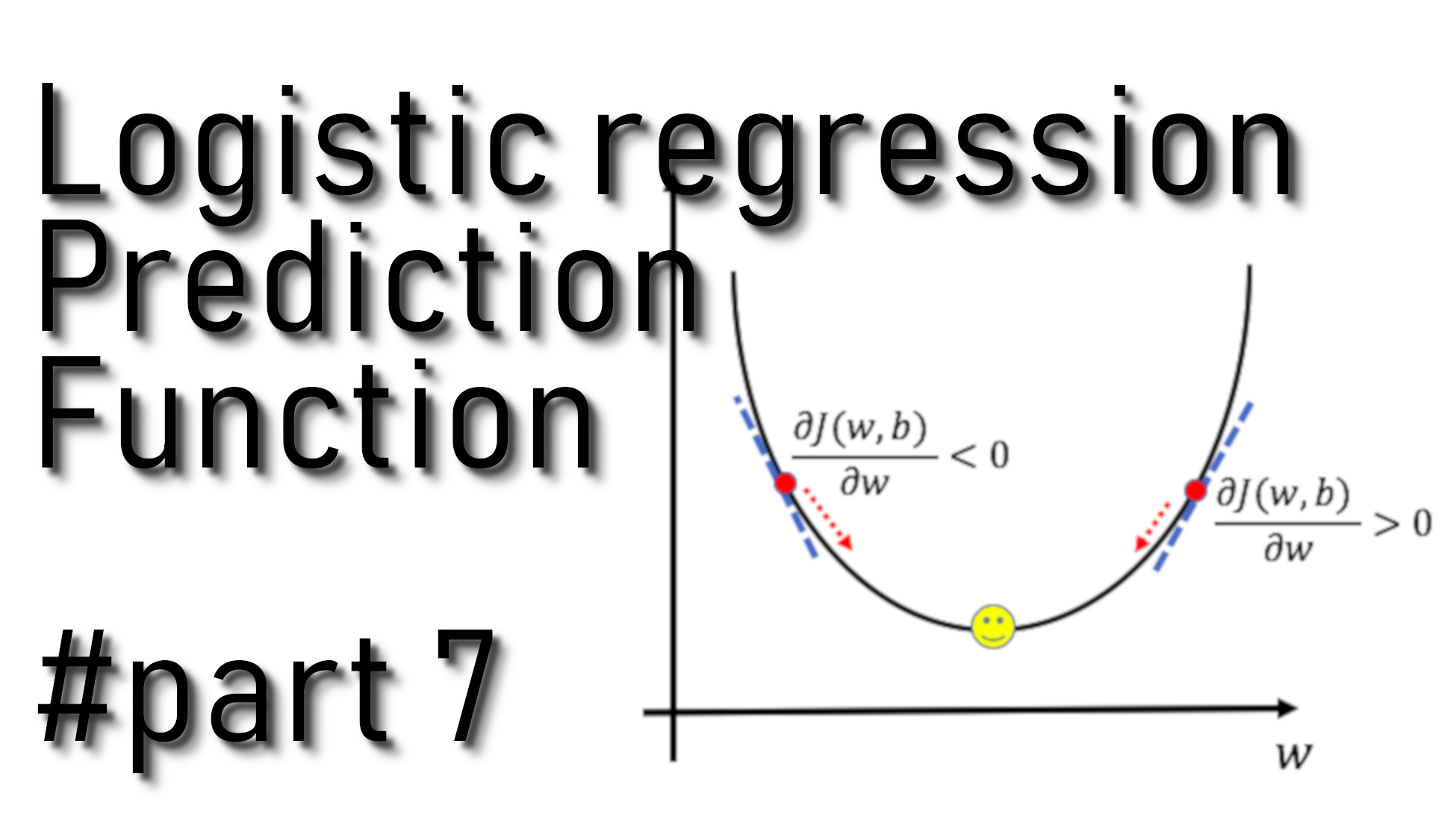# Logistic Regression predict function

## Logistic regression predict function

In the previous tutorial, we wrote an optimization function that will output the learned w and b parameters. Now we can use w and b to predict the labels for our dataset X. So, in this tutorial, we will implement the predict() function. There will be two steps computing predictions:

1. Calculate:
$\stackrel{^}{Y}=A=\sigma \left({W}^{T}X+b\right)$
2. Convert the entries of a into 0 (if activation <= 0.5 we'll get a dog) or 1 (if activation > 0.5 we'll get a cat). We will store the predictions in a vector 'Y_prediction'.

## Coding prediction function:

So we will implement a prediction function, but first, let's see what are the inputs and outputs to it:

Arguments:

w - weights, a NumPy array of size (ROWS * COLS * CHANNELS, 1);
b - bias, a scalar;
X - data of size (ROWS * COLS * CHANNELS, number of examples).

Return:

Y_prediction - a NumPy array (vector) containing all predictions (0/1) for the examples in X.

Here is the code:

``````def predict(w, b, X):
m = X.shape
Y_prediction = np.zeros((1, m))
w = w.reshape(X.shape, 1)

z = np.dot(w.T, X) + b
A = sigmoid(z)

for i in range(A.shape):
# Convert probabilities A[0,i] to actual predictions p[0,i]
if A[0,i] > 0.5:
Y_prediction[,[i]] = 1
else:
Y_prediction[,[i]] = 0

return Y_prediction``````

If we'll run our new function on previous values "predict(w, b, X)" we should receive the following results:

`predictions = [[1. 1. 0.]]`

From our results, we could say that we predicted two cats and one dog. But because input was not real images but just simple random test numbers, our predictions also don't mean anything.

## Full tutorial code:

``````import os
import cv2
import numpy as np
import matplotlib.pyplot as plt
import scipy

ROWS = 64
COLS = 64
CHANNELS = 3

TRAIN_DIR = 'Train_data/'
TEST_DIR = 'Test_data/'

#train_images = [TRAIN_DIR+i for i in os.listdir(TRAIN_DIR)]
#test_images =  [TEST_DIR+i for i in os.listdir(TEST_DIR)]

return cv2.resize(img, (ROWS, COLS), interpolation=cv2.INTER_CUBIC)

def prepare_data(images):
m = len(images)
X = np.zeros((m, ROWS, COLS, CHANNELS), dtype=np.uint8)
y = np.zeros((1, m))
for i, image_file in enumerate(images):
if 'dog' in image_file.lower():
y[0, i] = 1
elif 'cat' in image_file.lower():
y[0, i] = 0
return X, y

def sigmoid(z):
s = 1/(1+np.exp(-z))
return s

def propagate(w, b, X, Y):
m = X.shape

# FORWARD PROPAGATION (FROM X TO COST)
z = np.dot(w.T, X)+b # tag 1
A = sigmoid(z) # tag 2
cost = (-np.sum(Y*np.log(A)+(1-Y)*np.log(1-A)))/m # tag 5

# BACKWARD PROPAGATION (TO FIND GRAD)
dw = (np.dot(X,(A-Y).T))/m # tag 6
db = np.average(A-Y) # tag 7

cost = np.squeeze(cost)
"db": db}

w = np.array([[1.],[2.]])
b = 4.
X = np.array([[5., 6., -7.],[8., 9., -10.]])
Y = np.array([[1,0,1]])
'''
grads, cost = propagate(w, b, X, Y)
print(cost)

train_set_x, train_set_y = prepare_data(train_images)
test_set_x, test_set_y = prepare_data(test_images)

train_set_x_flatten = train_set_x.reshape(train_set_x.shape, ROWS*COLS*CHANNELS).T
test_set_x_flatten = test_set_x.reshape(test_set_x.shape, -1).T

print("train_set_x shape " + str(train_set_x.shape))
print("train_set_x_flatten shape: " + str(train_set_x_flatten.shape))
print("train_set_y shape: " + str(train_set_y.shape))
print("test_set_x shape " + str(test_set_x.shape))
print("test_set_x_flatten shape: " + str(test_set_x_flatten.shape))
print("test_set_y shape: " + str(test_set_y.shape))

train_set_x = train_set_x_flatten/255
test_set_x = test_set_x_flatten/255
'''
def optimize(w, b, X, Y, num_iterations, learning_rate, print_cost = False):
costs = []
for i in range(num_iterations):
grads, cost = propagate(w, b, X, Y)

# update w and b
w = w - learning_rate*dw
b = b - learning_rate*db

# Record the costs
if i % 100 == 0:
costs.append(cost)

# Print the cost every 100 training iterations
if print_cost and i % 100 == 0:
print ("Cost after iteration %i: %f" %(i, cost))

# update w and b to dictionary
params = {"w": w,
"b": b}

# update derivatives to dictionary
"db": db}

params, grads, costs = optimize(w, b, X, Y, num_iterations = 100, learning_rate = 0.009, print_cost = False)
'''
print("w = " + str(params["w"]))
print("b = " + str(params["b"]))
'''

def predict(w, b, X):
m = X.shape
Y_prediction = np.zeros((1, m))
w = w.reshape(X.shape, 1)

z = np.dot(w.T, X) + b
A = sigmoid(z)

for i in range(A.shape):
# Convert probabilities A[0,i] to actual predictions p[0,i]
if A[0,i] > 0.5:
Y_prediction[,[i]] = 1
else:
Y_prediction[,[i]] = 0

return Y_prediction

print(predict(w, b, X))	``````

## Conclusion:

Up to this point, now we know how to prepare our training data, how to optimize the loss iteratively to learn w and b parameters (computing the cost and its gradient, updating the parameters using gradient descent). And in this tutorial, we learned (w,b) to predict the labels for a given set of examples. So in the next tutorial, we'll merge all functions into a model, and we'll train it to predicts cats vs. dogs.